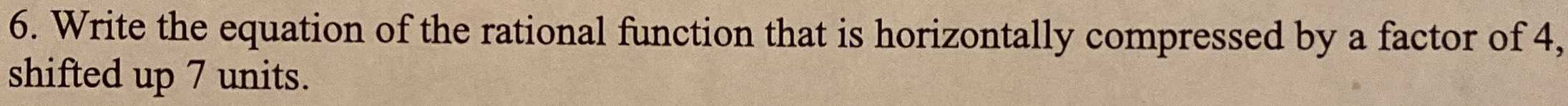### ¿Todavía tienes preguntas de matemáticas?

Pregunte a nuestros tutores expertos
Algebra
Pregunta6. Write the equation of the rational function that is horizontally compressed by a factor of $$4$$ , shifted up $$7$$ units.

$$f(x)\to f(4x)+ 7$$